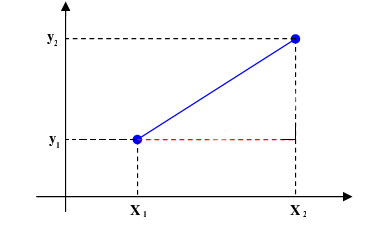# Distance Between Two Points Calculator

Created by Luciano Mino
Reviewed by Davide Borchia
Last updated: Jul 14, 2022

Our distance between two points calculator can quickly find the distance between any two points confined to a two-dimensional plane.

Within this short text, we will cover:

• How to find the distance between two points;
• How to use the distance between two points formula; and
• What is the shortest distance between two points.

Let's start!

Prefer watching rather than reading? Learn all you need in 90 seconds with this video we made for you:

## What is distance? Distance between two points definition

In its simplest definition, the distance between two points in a 2D plane is the length of the line segment connecting them.

For example, if we put into a graph the points $(0, 4)$ and $(4, 4)$, draw a line between them, and measure this line segment's length, we would get $4$ as a result.

This definition is derived from the Euclidean distance definition, and we can also define 1D, 3D, 4D, and any finite dimension Euclidean distance.

Of course, plotting and measuring lines anytime we want to find the distance between two points is not practical. That's where the distance between two points formula comes in.

## Distance between two points formula

We can obtain the mathematical distance definition from the Euclidean distance formula for a two-dimensional plane:

$d = \sqrt{(x_{2}-x_{1})^{2}+(y_{2}-y_{1})^{2}}$

where:

• $x_{1}$ and $y_{1}$ are the coordinates of any of the two points;
• $x_{2}$ and $y_{2}$ are the coordinates of the other point; and
• $d$ is the distance between them.

💡 This definition is what makes the shortest distance between two points on a two-dimensional plane always a line! Don't worry. We won't dwell deeper into math in this distance between two points calculator 😉.

## How do you find the distance between two points?

To find the distance between two points, simply follow these steps:

1. Find the XY coordinates of the first point (x₁, y₁). It doesn't matter which point we choose as long as we don't mix coordinates between them.
2. Find the XY coordinates of the other point (x₂, y₂).
3. Replace these values in the distance between two points formula: √[(x₂ - x₁)² + (y₂ - y₁)²].

## Other useful tools

If you enjoyed this distance between two points calculator and want to learn more about other distance definitions, check any of our other distance calculation tools:

• Distance;
• 2D distance;
• Length of a line segment;
• Coordinate distance; and
• Euclidean distance.

🙋 Give it a try! Type any two points' coordinates in the distance between two points calculator, and it will automatically output the distance between them.

## FAQ

### What is the shortest distance between two points?

The shortest distance between two points is a straight line connecting them. This definition only applies to flat surfaces or spaces. In a sphere, for example, the shortest distance between two points is an arc called great circle distance.

### What is the distance between (5, 10) and (8, 9)?

3.16228. We can find the distance between points (5, 10) and (8, 9) by replacing them in the distance between two points formula: √[(8 - 5)² + (9 - 10)²] = 3.16228.

Luciano MinoFirst point
x₁
y₁
Second point
x₂
y₂
Result
Distance
People also viewed…

### Body fat

Use the body fat calculator to estimate what percentage of your body weight comprises of body fat.

### Cramer's rule

The Cramer's rule calculator finds the solution to your system of equations and the values of the determinants used in the calculations.

### Rounding

Rounding tool allows you to round up or down any values.

### Sunbathing

Do you always remember to put on sunscreen before going outside? Are you sure that you use enough? The Sunbathing Calculator ☀ will tell you when's the time to go back under an umbrella not to suffer from a sunburn!